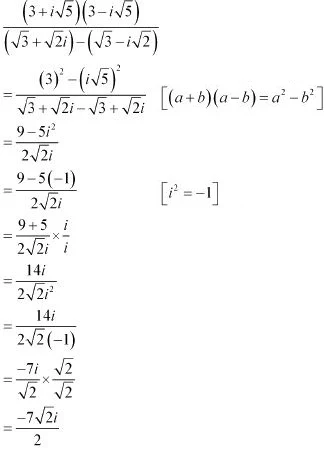# Class 11 Maths NCERT Solutions for Chapter 5 Complex Numbers and Quadratic Equations Exercise 5.1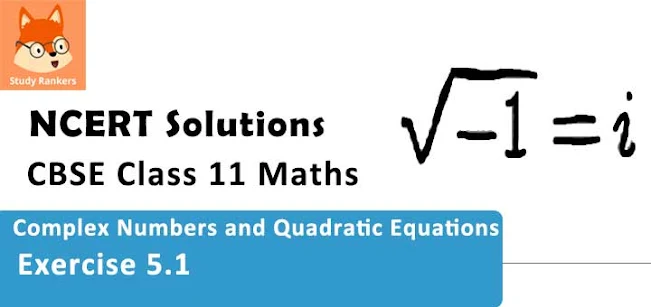### Complex Numbers and Quadratic Equations Exercise 5.1 Solutions

1. Express the given complex number in the form a + ib: (5i) (-3/5)i

Solution

(5i) (-3/5)i = -5 × (3/5) × i × i
= -3i2
= -3(-1)  [i2 = -1]
= 3

2. Express the given complex number in the form a + ib : i9 + i19

Solution

i9 + i19 = i4×2+1 + i4×4+3
= (i4)2. i + (i4)4. i3
= 1×i + 1×(-i[i4 = 1, i3 = -i]
= i + (-i)
= 0

3. Express the given complex number in the form a +ib : i-39

Solution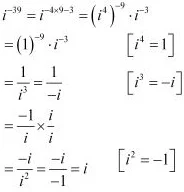4. Express the given complex number in the form a + ib : 3(7 + i7) + i(7 + i7)
Solution
3(7 + i7) + i(7 + i7) = 21 + 21i + 7i + 7i2
= 21 + 28i + 7 × (-1)  [∵i2  = -1]
= 14 + 28i

5. Express the given complex number in the form a + ib: (1 – i) – (–1 + i6)
Solution
(1 - i) - (-1 + i6) = 1 - i + 1 - 6i
= 2 - 7i

6. Express the given complex number in the form a + ib: (1/5 +i 2/5) - (4 + i 5/2)
Solution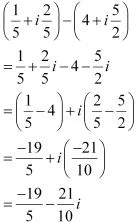7. Express the given complex number in the form a + ib: [(1/3 + 7/3) + (4 + i 1/3)] - (-4/3 + i
Solution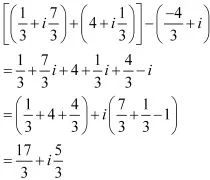8. Express the given complex number in the form a + ib : (1 - )4
Solution
(1 -i)2 = [(1 - i)2]2
= [12 + i2 + 2i2]2
= [1 - 1 - 2i]2
= (-2i)2
= (-2i)×(-2i)
= 4i2 = -4  [i2  = -1]

9. Express the given complex number in the form a + ib : (1/3 + 3i)3
Solution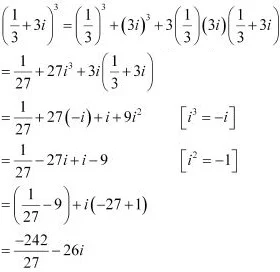10. Express the given complex number in the form a + ib : [-2 - (1/3)i]3
Solution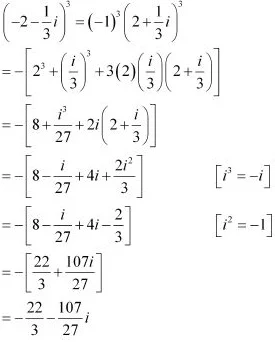11. Find the multiplicative inverse of the complex number 4 - 3i
Solution
Let z = 4 - 3i
Then, z  = 4 + 3i and |z|2 = 42 + (-3)2 = 16 + 9 = 25
Therefore, the multiplicative inverse of 4 - 3i is given by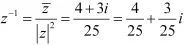12. Find the multiplicative inverse of the complex number √5 + 3i
Solution
Let z = √5 + 3i
Then, z = √5 + 3i and |z|2 = (√5)2 + 32 = 5 + 9= 14
Therefore, the multiplicative inverse of √5 + 3i is given by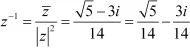13. Find the multiplicative inverse of the complex number –i
Solution
Let z = -i
Then, z  = i and |z|2 = 12 = 1
Therefore, the multiplicative inverse of -i is given by
z-1 = z /|z|2 = i/1 = i

14. Express the following expression in the form of a + ib.
[(3 + i√5)(3 - i√5)]/[(√3 + √2i)- (√3 - i√2)]
Solution